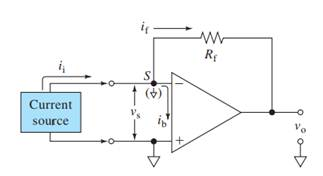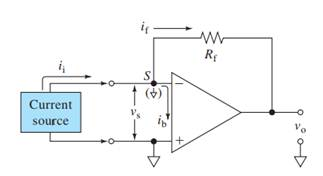# (a) Interpretation: Current follower that will produce a 1.0 V output for 10.0 µA input current should be designed. Concept introduction: Operational amplifiers can be used to measure or process currents by connecting them in the current follower mode. This mode provides a nearly zero resistance load to the current source and prevents it from being loaded by a measuring device or circuit. Here, V + , V - = input voltages V s = Input difference voltage V 0 = output voltage i b = input bias current i f = feedback current i i = input current R f = feedback resistor Also, R f = V 0 i i### Principles of Instrumental Analysis

7th Edition
Douglas A. Skoog + 2 others
Publisher: Cengage Learning
ISBN: 9781305577213### Principles of Instrumental Analysis

7th Edition
Douglas A. Skoog + 2 others
Publisher: Cengage Learning
ISBN: 9781305577213

#### Solutions

Chapter 3, Problem 3.8QAP
Interpretation Introduction

## (a)Interpretation:Current follower that will produce a 1.0 V output for 10.0 µA input current should be designed.Concept introduction:Operational amplifiers can be used to measure or process currents by connecting them in the current follower mode. This mode provides a nearly zero resistance load to the current source and prevents it from being loaded by a measuring device or circuit.Here,V+, V- = input voltagesVs = Input difference voltageV0 = output voltageib = input bias currentif = feedback currentii = input currentRf = feedback resistorAlso,Rf=V0ii

Interpretation Introduction

### (b)Interpretation:Effective input resistance of the current follower designed in part (a) should be calculated.Concept introduction:Here,V+, V- = input voltagesVs = Input difference voltageV0 = output voltageib = input bias currentif = feedback currentii = input currentRf = feedback resistorRi = effective input resistanceA = open loop gainAlso,Ri=RfA

Interpretation Introduction

### Want to see the full answer?

Check out a sample textbook solution.

### Want to see this answer and more?

Experts are waiting 24/7 to provide step-by-step solutions in as fast as 30 minutes!*

*Response times may vary by subject and question complexity. Median response time is 34 minutes for paid subscribers and may be longer for promotional offers.